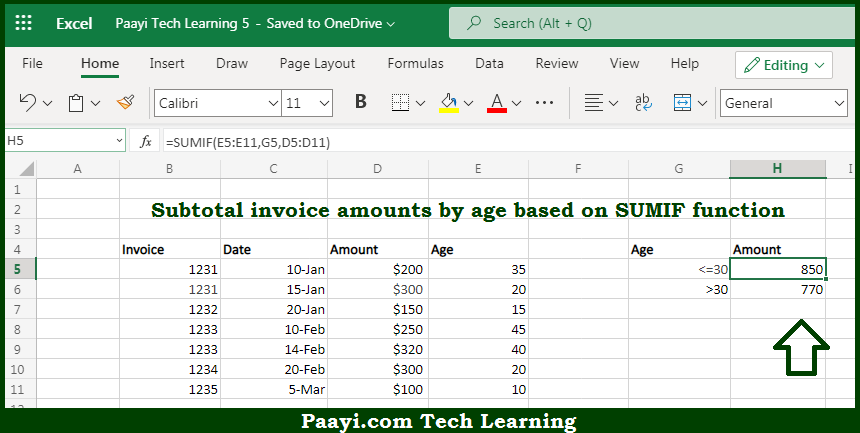# Learn How to SUBTOTAL Invoices By Age in Microsoft Excel

Written by | 0 Comments | 623 Views

In this article, you will learn how to COUNT various things in Microsoft Excel using a single/combination(s) of functions. You will also know how to SUBTOTAL Invoices By Age and see the generic formula.

SUBTOTAL Invoices By Age in Microsoft Excel

The main purpose of this formula is to subtotal invoice amounts by age. Here we will learn how to subtotal the provided invoices easily by age in Microsoft Excel. That implies, with the help of a formula based on the  SUMIF function you can able to subtotal invoice amounts by age. So, with the help of this formula, you can able to subtotal the provided invoices easily by age in Microsoft Excel.

General Formula to SUBTOTAL Invoices By Age

=SUMIF(age,criteria,amount)

The Explanation for the SUBTOTAL Invoices By AgeSo we know that with the help of the given formula above you can able to subtotal values by invoice number. Here we will learn how to subtotal the provided values easily in Microsoft Excel. As we know that the SUMIF function takes three arguments which are - range, criteria, and sum-range. In the example given above, we are using Range: the range of cells (E5:E11) to which criteria in column G is applied. Criteria: G5 - a reference to the adjacent cell in column G, which contains the criteria. This is a relative address so that it will change when copied down. So, with the help of this formula, you can able to able to subtotal values by invoice number.